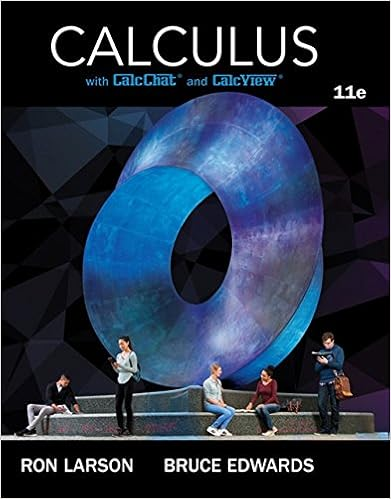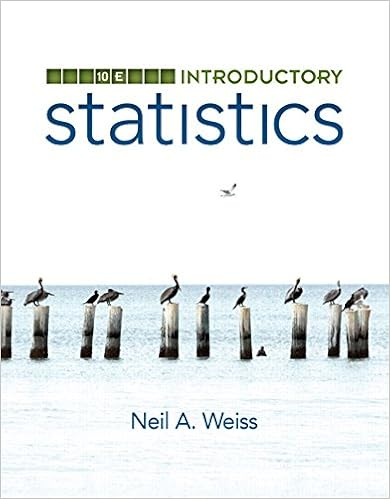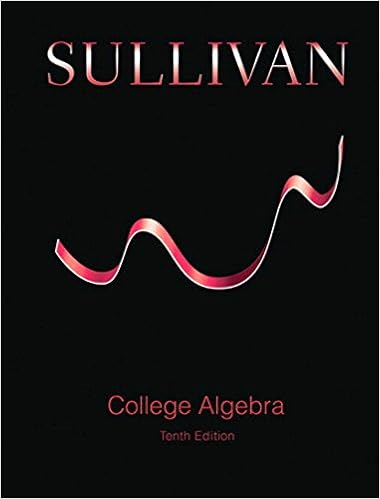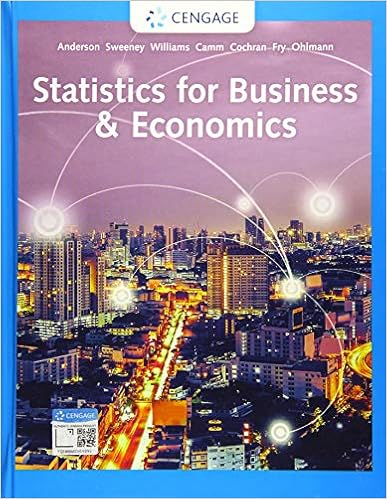# Final-2012.pdf - SECTION: NAME: CBE 320 Transport Phenomena...

• Test Prep
• 10
• 100% (5) 5 out of 5 people found this document helpful

This preview shows page 1 - 4 out of 10 pages.

SECTION:NAME:CBE 320May 15, 2012Transport PhenomenaFinal ExamPart A: Short Answer1.(5 pts.) What are thedimensionsof the following quantities?a.JstarAb.NAc.DABd.kx(mass transfer coefficient)e.cA2.(5 pts.) A magnetohydrodynamic pump can drive a fluid by exerting a body forceKH2([=] force/volume)on the fluid, whereKis a material parameter, andHis the magnetic field strength.Assuming the fluid isincompressible and Newtonian with constantρandμ, write down the equation of motion for this situation indifferential form. Recall that the Navier-Stokes equation isρDvDt=-∇p+μ2v+ρg1
##### We have textbook solutions for you!The document you are viewing contains questions related to this textbook.
Chapter 15 / Exercise 18
Calculus
Edwards/LarsonExpert VerifiedBrowse all Textbook Solutions
3.(5 pts.) In a certain problem, the diffusivity is related to the mass transfer rate byDAB=NAz|z=z1RTΔzpln(pB2/pB1)CalculateDABin units of m2/s for the following parameter values:NAz|z=z1= 7.26×10-9g-mole/cm2·s,R= 8.31451J/mole·K,T= 273K,Δz= 17.1cm,p= 755mm Hg,pB2= 755mm Hg, andpB1=722mm Hg. The following information may be useful:1m=100cm760mm Hg=1atm1atm=101.325kPa1Pa=1N/m21J=1N·m1mole=1g-mole4.(5 pts.) Write down the Stefan-Boltzmann law. Define each quantity and give their SI (i.e., MKSA) units.2
5.(5 pts.) GasAdissolves in a beaker of stagnant liquidB, and reacts withBto formC(RA=-kprimeprimeprime1cA).Sketchplots ofcAas a function ofzfor both small and largekprimeprimeprime1. Here,zis the distance below the liquid/gas interface.6.(5 pts.) A material (A) is diffusing (no convection) into a porous cylinder (radiusR, lengthL) from a flowingfluid (B). The concentration of speciesAwithin the fluidiscA(r, θ, z, t), and the diffusivity within the fluidisDAB. Using this information, write an integral expression for the total rate of transport of speciesAfromthe fluid into the cylinder (molesA/time). You may assume thatAis dilute so that the total concentration isconstant, and that transport at the cylinder ends may be neglected.3

Course Hero member to access this document

Course Hero member to access this document

End of preview. Want to read all 10 pages?

Course Hero member to access this document

Term
Fall
Professor
Staff
Tags
##### We have textbook solutions for you!
The document you are viewing contains questions related to this textbook.The document you are viewing contains questions related to this textbook.
Chapter 15 / Exercise 18
Calculus
Edwards/LarsonExpert Verified
•••